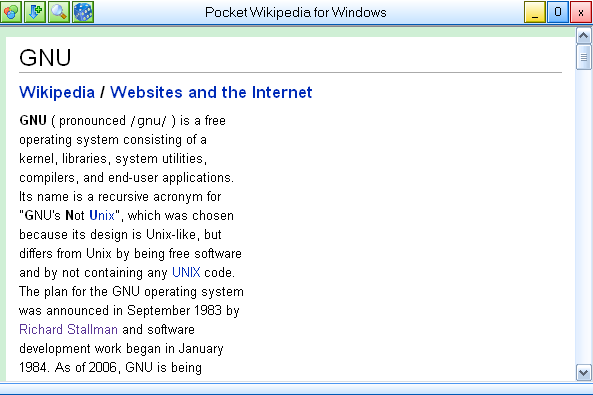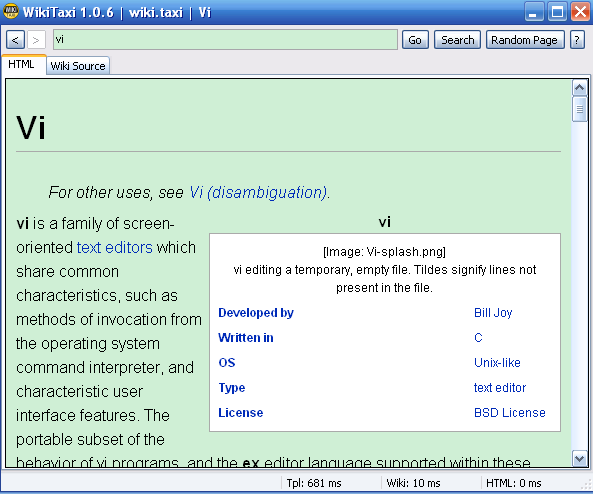CraneSearch
Loading## C语言有点变态

#include<stdio.h>
int f(int x)
{
printf("%d\n",x);
}
int main(void)
{
int (*pf)(int);
pf=f;   //正常用法
pf(5);
(**pf)(5);      //这是什么
(****************f)(5)//这个变态啊
pf=&f;  //这个也没问题
pf(6);
pf=*****f;      //这是干什么
pf(7);
system("pause");
return 0;
}

## VHDL编程之可逆计数器

library ieee;
use ieee.std_logic_1164.all;
use ieee.std_logic_unsigned.all;
use ieee.std_logic_arith.all;
entity counte is
port(cp,clr,ld,m:in std_logic;
abcd:in std_logic_vector(3 downto 0);
qcc:out std_logic;
qcount:out std_logic_vector(3 downto 0));
end;
architecture count of counte is
begin
process(cp,clr,ld)
begin
if(clr='0')then
qcount<="0000";
if(m='0')then
qcc<='0';
end if;
elsif(ld='0')then
if(abcd="0000" and m='0')then
qcount<=abcd;
qcc<='0';
elsif(abcd="1111" and m='1')then
qcount<=abcd;
qcc<='0';
else
qcount<=abcd;
qcc<='1';
end if;
elsif(cp'event and cp='1')then
if(qcount="1110" and m='1')then
qcount<="1111"
qcc<='0';
elsif(m='1')then
qcount<=qcount+1;
qcc<='1';
end if;
if(qcount="0001" and m='0')then
qcount<="0000";
qcc<='0';
elsif(m='0')then
qcount<=qcount-1;
qcc<='1';
end if;

end if;
end process;
end  count;

## WikiTaxi--随身带的wikipediaWikiTaxi，也是一个移动版的，不需要安装，只有两个可执行文件，一个是主程序，一个用来导入数据库，只有有了个这个数据库才能做到在本地查询，这个数据库可以到wikipedia上去下，英文数据库的地址在这里，这个是最latest的版本，bz2压缩的，大概有4.8G的大小，可以想像里面有多少内容，你也可以从朋友那里拷。有了这个后用那个包中的WikiTaxi Importer把这个xml.bz2导入成WikiTaxi database文件（以.taxi为扩展名）。PS：这个可能用的时间比较长。## 比较程序的效率

``` ```

``` 编写程序，测试两条循环语句的执行时间。分析为何执行时间不同。   int A[ROWS][COLS]; for(row=0; row<ROWS;row++)     for(col=0;col<COLS;col++)        A[row][col]=0; for(col=0;col<COLS;col++)     for(row=0; row<ROWS; row++)        A[row][col]=0; ```
``` ```

clock_t clock( void );

#ifndef _CLOCK_T_DEFINED
typedef long clock_t;
#define _CLOCK_T_DEFINED
#endif

#define CLOCKS_PER_SEC ((clock_t)1000)

1. #include<stdio.h>
2. #include<time.h>
3. #include<stdlib.h>
4. #define ROWS 10000
5. #define COLS 10000
6. int a[ROWS][COLS];
7. main()
8. {
9.         clock_t start,finish;
10.         double elapsed1,elapsed2;
11.         int row,col;
12.         start=clock();
13.         for(row=0;row<ROWS;row++)
14.         for(col=0;col<COLS;col++)
15.         a[row][col]=0;
16.         finish=clock();
17.         elapsed1=(double)(finish-start)/CLOCKS_PER_SEC;
18.         start=clock();
19.         for(col=0;col<COLS;col++)
20.         for(row=0;row<ROWS;row++)
21.         a[row][col]=0;
22.         finish=clock();
23.         elapsed2=(double)(finish-start)/CLOCKS_PER_SEC;
24.         printf("the first loop cost %f seconds.\n",elapsed1);
25.         printf("the second loop cost %f seconds.\n",elapsed2);
26.         system("pause");
27.         return 0;
28. }

the first loop cost 1.381000 seconds.
the second loop cost 6.880000 seconds.

## C/C++中的日期和时间

time_t time(time_t * timer);

#ifndef _TIME_T_DEFINED
typedef long time_t;         /* 时间值 */
#define _TIME_T_DEFINED      /* 避免重复定义 time_t */
#endif

char * ctime(const time_t *timer);

Tue Jan 19 11:14:07 2038

#ifndef _TM_DEFINED
struct tm {
int tm_sec;     /* 秒 – 取值区间为[0,59] */
int tm_min;     /* 分 - 取值区间为[0,59] */
int tm_hour;    /* 时 - 取值区间为[0,23] */
int tm_mday;    /* 一个月中的日期 - 取值区间为[1,31] */
int tm_mon;     /* 月份（从一月开始，0代表一月） - 取值区间为[0,11] */
int tm_year;    /* 年份，其值等于实际年份减去1900 */
int tm_wday;    /* 星期 – 取值区间为[0,6]，其中0代表星期天，1代表星期一，以此类推 */
int tm_yday;    /* 从每年的1月1日开始的天数 – 取值区间为[0,365]，其中0代表1月1日，1代表1月2日，以此类推 */
int tm_isdst;   /* 夏令时标识符，实行夏令时的时候，tm_isdst为正。不实行夏令时的进候，tm_isdst为0；不了解情况时，tm_isdst()为负。*/
};
#define _TM_DEFINED
#endif

time.h还提供了两种不同的函数将日历时间（一个用time_t表示的整数）转换为我们平时看到的把年月日时分秒分开显示的时间格式tm：

struct tm * gmtime(const time_t *timer);
struct tm * localtime(const time_t * timer);

char * asctime(const struct tm * timeptr);

1. #include "time.h"
2. #include "stdio.h"
3. int main(void)
4. {
5.   time_t lt;
6.   struct tm st,*pt;
7.   lt=time(NULL);
8.   printf("The Calendar time now is %ld\n",lt)
9.   lt =2147483647;
10.   printf("The bug time(local) is %s\n",ctime(&lt));
11.   pt=gmtime(&lt);
12.   printf("The bug time(UTC) is %s",asctime(pt));
13.   pt=localtime(&lt);
14.   printf("The bug time(local) is %s\n",time(pt));
15.   system("pause");
16.   return 0;
17. }

The Calendar time now is 1239709783
The bug time(local) is Tue Jan 19 11:14:07 2038

The bug time(UTC) is Tue Jan 19 03:14:07 2038
The bug time(local) is Tue Jan 19 11:14:07 2038

size_t strftime(
char *strDest,
size_t maxsize,
const char *format,
const struct tm *timeptr
);

%a 星期几的简写
%A 星期几的全称
%b 月分的简写
%B 月份的全称
%c 标准的日期的时间串
%C 年份的后两位数字
%d 十进制表示的每月的第几天
%D 月/天/年
%e 在两字符域中，十进制表示的每月的第几天
%F 年-月-日
%g 年份的后两位数字，使用基于周的年
%G 年分，使用基于周的年
%h 简写的月份名
%H 24小时制的小时
%I 12小时制的小时
%j 十进制表示的每年的第几天
%m 十进制表示的月份
%M 十时制表示的分钟数
%n 新行符
%p 本地的AM或PM的等价显示
%r 12小时的时间
%R 显示小时和分钟：hh:mm
%S 十进制的秒数
%t 水平制表符
%T 显示时分秒：hh:mm:ss
%u 每周的第几天，星期一为第一天 （值从0到6，星期一为0）
%U 第年的第几周，把星期日做为第一天（值从0到53）
%V 每年的第几周，使用基于周的年
%w 十进制表示的星期几（值从0到6，星期天为0）
%W 每年的第几周，把星期一做为第一天（值从0到53）
%x 标准的日期串
%X 标准的时间串
%y 不带世纪的十进制年份（值从0到99）
%Y 带世纪部分的十进制年份
%z，%Z 时区名称，如果不能得到时区名称则返回空字符。
%% 百分号

1. #include <stdio.h>
2. #include <time.h>
3.
4. main( void )
5. {
6.   struct tm *newtime;
7.   char tmpbuf;
8.   time_t lt1;
9.   time( &lt1 );
10.   newtime=localtime(&lt1);
11.   strftime( tmpbuf, 128, "Today is %A, day %d of %B in the year %Y.\n", newtime);
12.   printf(tmpbuf);
13.   system("pause");
14. }
15.

Today is Tuesday, day 14 of April in the year 2009.